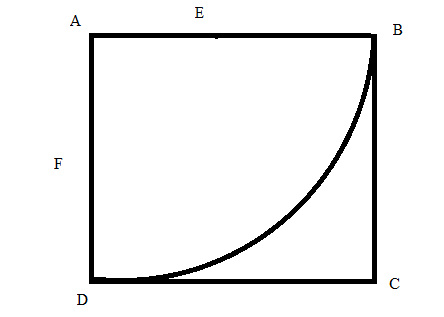# The maximum and minimum areas that the triangle AEF can have.### Single Variable Calculus: Concepts...

4th Edition
James Stewart
Publisher: Cengage Learning
ISBN: 9781337687805### Single Variable Calculus: Concepts...

4th Edition
James Stewart
Publisher: Cengage Learning
ISBN: 9781337687805

#### Solutions

Chapter 4, Problem 18P
To determine

## The maximum and minimum areas that the triangle AEF can have.

Expert Solution

### Explanation of Solution

Given information:

ABCD is a square piece of paper with sides of length 1m. A quarter circle is drawn from B to D with center A. The piece of paper is folded along the EF, with E on AB and F on AD so that A falls on the quarter circle.

Calculations:

Here according to the given information we draw the diagram.Now E can be anywhere on AB and F can be anywhere on AD. Now Area of the triangle AEF is,

Area of ΔAEF=12AE×AF.   Since ΔABD is a right angle triangle and A=90°.

Now the area of the triangle will be maximum if E is very close to B and F is very close to D.

Also the area of the triangle will be minimum if E is very close to A and F is very close to A.

Therefore maximum area of the triangle is,